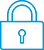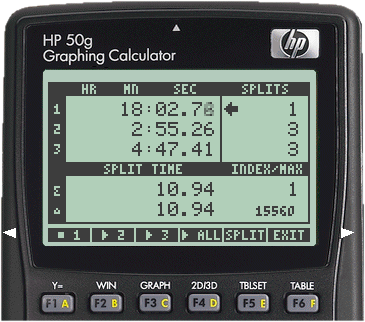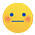cancel
Showing results for
Search instead for
Did you mean:It has been a while since anyone has replied. Simply ask a new question if you would like to start the discussion again.New member
3 1 0 0
Message 1 of 9
1,286
Flag Post

# HP12C calculator bond price special caseHP Recommended

Hi all!

There's a specific scenario I'm trying to solve for a bond price:

Stellar Corp. recently issued \$100 par value deferred coupon bonds, which will make no coupon payments in the next four years. Regular annual coupon payments at a rate of 8% will then be made until the bonds mature at the end of 10 years. If the bonds are currently priced at \$87.00, their yield to maturity is closest to:

1. 6.0%.
2. 8.0%.
3. 10.1%.

Answer is 6.0%.

Is there a way for this whole scenario to be calculated with the TVM keys and of the HP12C?

8 REPLIES 8Level 6
140 139 29 60
Message 2 of 9
Flag PostHP Recommended

Classify the following as an "educated guess", because I know that bond calculations can be tricky.  I believe this one has been set up in such a way that some of the trickier parts have been mitigated, but I'm not an expert and you should consider the following more as a possibility than absolute fact.  Hopefully others with more knowledge of the treatment of bonds will speak up and correct this if needed.

Knowing the specific items identified in the problem description, it appears to me that you can translate this problem into a fairly straightforward TVM scenario with known values for n, PV, and FV.

n: 10 (years)

PV: -87 (the purchase price of the bond, negative for outward cash flow)

FV: this is where the fun comes in.  You have to understand the terminology to make any sense of this.  FV will be the redemption value of the bond, which in this case is the par value (\$100 principal) + any coupon payments (\$8 x 6).  So this makes FV = (100 + 48) = 148.

Try the following:

- Clear FIN registers
- 10 n
- -87 PV
- 148 FV
- i

The computed effective annual rate is 5.4567%, which is closest to 6 in the problem description.Level 6
305 297 32 76
Message 3 of 9
Flag PostHP Recommended

I am not an expert either but I think we can even improve this by also taking the interest on the coupon payments into account.

You could not have done that because you did not know yet what the interest was, but now we have a first approximation.

With this interest rate, so 5.46%, we can now calculate the value of the coupon payments after 10 years.

This is a TVM calculation with:

n=6

i=5.46

PV=0

PMT=8

and we have to solve for FV, which gives: 55.05 instead of 48.

Again we have to add this to 100, which gives 155.05

When we next solve again for i with

n=10

PV=87

FV=-155.05

PMT=0

we get : i=5.95, which is already very close to 6

We can continue this and get closer and closer to the interest rate, which in these calculations is identical to the 'yield to maturity'.Level 6
140 139 29 60
Message 4 of 9
Flag PostHP Recommended

@Jan_D wrote:

...I think we can even improve this by also taking the interest on the coupon payments into account.

You could not have done that because you did not know yet what the interest was, but now we have a first approximation....

We can continue this and get closer and closer to the interest rate, which in these calculations is identical to the 'yield to maturity'.

That seems reasonable, and it takes into consideration the underlying assumption of interest payments being reinvested (which seems to be part of the definition of YTM, even if that interest isn't actually paid out).  But it's possible we may both be off a bit.  This reference seems to be somewhat close to the original question, and could possibly be made to fit for years 5-10.

If I'm understanding the method used in that linked article from the tvmcalcs site, the following TVM parameters would apply to years 5-10:

n: 6 (years remaining after 1-4)

PV: -87 (the value at the beginning of year 5)

PMT: 8 (END mode assumed)

FV: 100 (the par value)

Solve for i: 11.07997857

At this point YTM (i) is known for the 6-year period where coupons apply.  We now need to translate that to the full 10-year period.  Let's start by determining the FV of \$87 at that same interest rate for 6 years, but with no payments:

PMT: 0

Solve for FV: 163.4308263

Now convert the effective interest rate from 6 years to 10:

n: 10

Solve for i: 6.507814296

Of course all of the above could be wrong as well.Hopefully someone who knows bond calculations better than us will chime in!Level 6
305 297 32 76
Message 5 of 9
Flag PostHP Recommended

I like Avrora's question because it helps me to understand more about bonds.

I think it is good to explain why I think 6% is the correct answer to his question, because I have not really done this so far.

To understand this I ask myself: what would be a reasonable price for the bond in case of a stable market interest rate of 6%?

To determine this price we have to know the future payments, and there are 7 of them: 6 coupon payments of 8 dollars and a last payment of 100 dollars, so one could think a reasable price would be obtained by adding them all, which gives 148 dollar.

Of course this is not correct because  100 dollars in the future is worth less than 100 dollars now, so we have to add the present values of them all, and we could do this with TVM calculations, which would require 7 of them.

I have not done this but it would be a good exercise to do it and I am pretty sure that the result is very near to 87 dollars.

It is easier to follow another approach and that is to first calculate the future value of all payments, and after this convert this total future value to the present value.

The future value of the 6 coupon payments can be obtained by a TVM calculation with:

n=6

PMT=8

i=6

PV=0

which gives FV=55.80255

We have to add this to the last payment of 100, which gives: 155.80255

Next we convert this back to the present value with a TVM calculation with:

n=10

PMT=0

i=6

FV=155.80255

which gives PV=86.99933

This is very close to the price of the bond which is 87.

Because an interest rate of 6% is in accordance with 87  dollars being a reasonable price for the bond we call this the

'yield to maturity' of the bond when priced for 87 dollars (in my opinion)

The method described in messages 2 and 3 also leads to this result of 6%Level 6
140 139 29 60
Message 6 of 9
Flag PostHP Recommended

@Jan_D wrote:

I like Avrora's question because it helps me to understand more about bonds.

I think it is good to explain why I think 6% is the correct answer to his question, because I have not really done this so far.

I agree with everything you said, including the assertion that the actual YTM is 6% for this problem.  This has been a good exercise to learn more about bonds.

The more I experiment with the numbers in this case, the more I realize that my earlier estimation attempts could be thrown off fairly significantly with simple changes in the values given in the original problem description.  The deferred coupon payments make a simple TVM calculation impossible for this problem.  The only real way to calculate the actual YTM is through an iterative approach (similar to how the TVM functions determine i).

The fact that a multiple-choice answer is provided in this case makes the problem easier to assess, since you can simply try each one to see how closely it fits.  I've used a similar approach to yours above.  It first simply moves the purchase price to the beginning of year 5, then determines if the FV at maturity matches the stated par value when the coupon payments are added in as well.

As an example, let's try one of the wrong answers to see if it fits (8%):

4 n
8 i
-87 PV
0 PMT
Solve for FV: 118.3625395
...which then becomes the PV in the next calculation:
CHS PV
6 n
8 PMT
Solve for FV: 129.1390424, which is clearly not close to the stated par value of 100.

Now let's check 6%...

4 n
6 i
-87 PV
0 PMT
Solve for FV: 109.8354955
...which then becomes the PV in the next calculation:
CHS PV
6 n
8 PMT
Solve for FV: 100.0012013, which is as close to the par value of 100 as we need to be to verify this result.

If we had simply been asked to determine the YTM without any hints to the correct value, an iterative approach that refines YTM (i) can be used.  It took 6 iterations when I tried the original problem to converge to 6%.  It's tedious, but it can be done.Level 6
305 297 32 76
Message 7 of 9
Flag PostHP Recommended

The tvmcalcs site you linked to gives an easy method to determine the YTM for bonds with periodic payments, using a TVM calculation, and you gave a correct example of it.

At first I did not understand how this could give the right answer and considered it as an ingenious trick.

I still consider it ingenious but I now realize that it is not difficult to understand once you know which method you have to apply.Level 6
140 139 29 60
Message 8 of 9
Flag PostHP Recommended

Taking the above factors into consideration, let's change the perspective slightly and illustrate how this problem could be solved with different goals in mind.

Perspective 1: The seller of the bond wants to price it appropriately for an assumed yield to maturity of 8.500%.  What would the new price be?  Assume that all of the other parameters are the same as previously given.

Two TVM calculations are needed, due to the deferred coupon payments.  Working backwards from par value, we first calculate the PV at the beginning of year 5:

6 n
8.5 i
8 PMT
100 FV
Solve for PV: -97.72320642

That value now becomes the FV for the second calculation, which is a standard compound interest scenario:

CHS FV
0 PMT
4 n
Solve for PV: -70.51455273
So the purchase price would now be \$70.51

Perspective 2: The purchaser is weighing different options, and doesn't know the actual yield to maturity of the bond being offered.  How can that value be determined?

This is where things get more interesting.  We can't do a simple TVM calculation due to the coupon deferral.  So we have to use what some sources refer to as a "trial and error" method to come up with a reasonable estimate (for simplicity I'll limit to 3 decimal places here).

I'm sure there are multiple ways to approach this, and I'll describe one approach based on what we've already determined from above.  I'll start with a guess at the YTM based on the coupon rate, then refine it successively until the rounded value appears to converge.

First, calculate the FV of the bond at the completion of year 4 with an assumed rate of 8%:

4 n
8 i
-70.51 PV
0 PMT
Solve for FV: 95.92807657

If 8% is the correct YTM, then we should be able to "back into it" by calculating a new i with all of the other known values:

CHS PV (the previous FV is now the current PV)
6 n
8 PMT
100 FV
Solve for i: 8.905146793

The new i is not the same as the previous (rounded to 3 decimal places), so I average them (8.452573395) and repeat the process.  Each successive attempt results in the following rounded values for i:

8.453
8.496
8.500
8.501
8.501

It appears that the YTM has converged.  So we now know that, with a purchase price of \$70.51, the YTM of the bond is about 8.501%.  Why did it not converge to 8.500%?  Because the purchase price was rounded down from the more accurate value of 70.51455273.  Starting with a slightly smaller purchase price required a slightly larger YTM to arrive at the final par value.Level 6
305 297 32 76
Message 9 of 9
Flag PostHP Recommended

On the HP12c Avrora's question can be solved much easier, not with the help of the TVM keys but with the use of cash flows (diagrams) and the function keys NPV (net present value) and IRR (internal rate of return).

How to deal with cash flows is explained on page 57 and following of the User Guide:

http://www.calculatrices-hp.com/uploads/pdf_en/12c_users_guide_English.pdf

First we want to determine the price for the bond at interest rates 6.0%, 8.0% and 10.1%

The cash flow of the bond consists of the payments:

An initial payment of 0.

4 subsequent payments of 0

Another 5 payments of 8

A final payment of 100+8=108.

Now we are going to enter these payments into the calculator.

First clear all registers by pressing  f-CLEAR-REG.

Next:

0  g-CF0 (store 0 into cash flow register 0)
0  g-CFj followed by 4 g-Nj (store 0 into cash flow register1 and there are 4 subsequent payments)
8  g-CFj followed by 5 g-Nj (store 8 into cash flow register 2 and there are 5 subsequent payments)
108  g-CFj (store 108 into cash flow register 3).

We now want to calculate the sum of their present values at an interest rate of 6%.
We enter 6 i and press f-NPV and get 86.99933
For an interest rate of 8% we enter 8 i, next f-NPV and we get 73.503
For an interest rate of 10.1% we enter 10.1 i, next f-NPV and we get 61.848

The interest rate in these calculations is the same as the YTM (yield to maturity) of the bond and the sum of the present values is the PRICE of the bond, so we see that the price for 6% is by far closest to 87, so answer 1 is corrrect.

Instead of trying several interest rates and calculate the corresponding NPV which is the price, we could also easily have calculated the interest rate directly, given a PRICE of 87.

Before going further we press: RCL  n which returns 3.

This is the number of cash flow registers we entered, apart from the initial one.

We now are going to change our cash flows a little to account for the initial price of 87.

We consider this as an initial investment, so the cash flow diagram remains the same apart from an initial payment of -87 instead of 0.

For this cash flow diagram we are going to solve IRR, which is the same as the YTM of a bond.

It is the same because it calculates the interest rate for which the net present value is 0, and because the

net present value  equals  -87  + NPV(payments of the bond)  this implies that NPV(payments of the bond)=87, and this is what we want.

We set the initial payment to -87 by:

87 CHS g-CF0

When we now press RCL n again we get 0 instead of 3 because the calculator thinks that we start another cash flow diagram.

However, we want to conserve our earlier inputs for the cash flow registers and we set n to 3 again: 3 n

Now we press f-IRR  which returns 5.99991 and we got the YTM  for a price of 87.

† The opinions expressed above are the personal opinions of the authors, not of HP. By using this site, you accept the Terms of Use and Rules of Participation